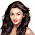# Hacker Rank solution for Lower Bound-STL In C++

Problem:- Lower Bound-STL Hacker Rank Solution or Lower Bound-STL hacker rank solution c++ orMulti Level Inheritance in java or Lower Bound-STL solution or Lower Bound-STL solution in C++ or Lower Bound-STL hacker rank solution in Java or Lower Bound-STL hacker rank solution c++ or Lower Bound-STL hacker rank solution c++ or Lower Bound-STL program in c or Lower Bound-STL solution in C or Hacker Rank Solution For Lower Bound-STL in C++ or Lower Bound-STL in C++ or Hacker Rank solution for Lower Bound-STL in C++.

Logic:- So basically is this Lower Bound-STL(Lower bound is a function that can be used with a sorted vector.) problem an array is given and number of query Q(an integer) is given we have to find that if a Query is present in an array then program returns an index of a number with "Yes" without quotes and if the query is not present then it should return "No" without quotes and also number greater than query. If you do not get don't worry I am here let's take an example.

Explanation:-

Sample Input

8                          // Array Size
1 1 2 2 6 9 9 15  // Array elements
4                         // Number of Query
1                         // Query 1
4                         // Query 2
9                         // Query 3
15                        // Query 4

Sample Output

Yes 1
No 5
Yes 6
Yes 8

So according to first query 1 is present in an index 1 so our output is Yes 1, Now move on to second query 4 is not present in array that's why the output is No 5, according to condition if number is not present in an array then it should return a next greater number than query. Now next query is 9 it is present in an index 6 so the output is Yes 6. and the last query is 15 it is also present in an array that's why the output is Yes 8.

Note:- Here an array index is starting with 1, Normally start with 0.

Check- Geeksforgeeks solution for School, Basic, Easy, Medium, Hard Domain.

Copy the full solution or colored code and paste into the hacker rank editor and click to Run Code if the code runs successfully then click to Submit Code. Before pasting the code into the editor make sure you have chosen c++ editor in the top right drop down option.

Solution:-

#include <cmath>
#include <cstdio>
#include <vector>
#include <iostream>
#include <algorithm>
using namespace std;

int main()
{
int m, num;
cin >> m;
vector<int> v;
for (int i=0; i<m; i++)
{
cin >> num;
v.push_back(num);
}
int n, val;
cin >> n;
for (int i=0; i<n; i++)
{
cin >> val;
vector<int>::iterator low = lower_bound(v.begin(), v.end(), val);
if (v[low - v.begin()] == val)
cout << "Yes " << (low - v.begin()+1) << endl;
else
cout << "No " << (low - v.begin()+1) << endl;
}
return 0;
}

Output:-

1.1.